## Welcome to the World of Modelling and Simulation

### What is Modelling?

This blog is all about system dynamics modelling and simulation applied in the engineering field, especially mechanical, electrical, and ...### A PID Controller Design by Relay Auto-tuning in SIMULINK

Part 01 (Designing the PID Controller by Relay Auto-tuning)

Ziegler–Nichols Rules for Tuning PID Controllers:
In this experiment, Ziegler-Nichols tuning method is explored to tune the PID controller for optimum system performance. Ziegler-Nichols gave two methods for selecting the proportional gain (Kp), integral time (Ti) and derivative time (Td) based on the transient response of the plant . In this report, the second method for controller tuning will be enlightened and implemented as well. Figure 1 shows the application of a PID controller in process control. According to Ziegler-Nichols’s  second method, at first the integral time is set to infinity and the derivative time is set to zero.Figure 1: A typical PID control system .

Then only using proportional control, the Kp value should be increased gradually from 0 until the system shows sustained oscillations and this value of the proportional control is known as critical value (Kcr). The desired controllers (P, PI, PD, and PID) are designed finding the critical gain Kcr and oscillation period Pcr from the experiment and then by using these experimental values to obtain appropriate gain parameters from table 1.

Table-1: Ziegler-Nichols Tuning Method Based on Critical GainThe objective of the lab is to implement a relay auto-tuner to find out the PID controller gain parameters. Implementation of the relay controller makes life easier than finding the critical gain values mentioned in the aforementioned excerpt. In this case, the amplitude of the relay auto-tuner is tuned until the system becomes marginally stable or oscillations centered at zero. Then, the amplitude of the auto-tuner is substituted in the following equation  to determine the ultimate gain.

(1)

Where h is the maximum or minimum amplitude from the nominal value zero and A is the amplitude of the system output signal. According to ; the following table is provided for relay auto-tuning.

Table-2: Ziegler-Nichols Tuning Rules When Relay Is ImplementedPu refers to the oscillation period of the close loop system.

Testing the PID Tuning for different Plants:

1. When the plant transfer function is
To implement this transfer function in SIMULINK, it is to be represented by matrix form. So, the numerator of the transfer function is  and denominator of the transfer function is [1 3 3 1].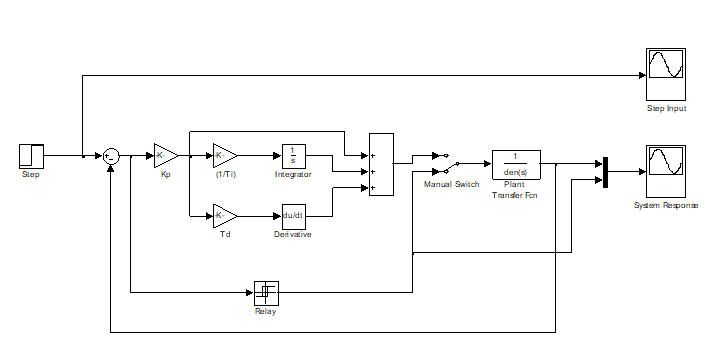Figure 2: SIMULINK model representing the application of auto-tuner to determine the ultimate gain.

The amplitude of the relay auto-tuner pulse signal h is 0.1 for which the system becomes marginally stable. And, the sustained oscillation of the plant is obtained by this relay auto tuner value. The ultimate gain Kcu according to equation (1) is 7.96. (A=0.016, Pu=3.73)

Following figure 3 highlights the close loop system response and the relay auto-tuner response from which the ultimate gain is determined. A manual switch is implemented to switch from relay auto-tuner mode to linear control mode (figure 2).Figure 3: Plot showing the close loop system dynamic response with the relay input.

From table 2, the tuning parameters for the P, PI, and PID controllers are calculated.

P Controller:

The gain value is 3.98. Figure (4) shows the SIMULINK model when P-controller is used. In addition, by using the gain parameter, the close loop system response to the step input is depicted in figure 5.

PI Controller:
The gain is 3.582 and integral time is 3.12.Figure 4: SIMULINK model representing the application of P controller to control the plant.Figure 5: Close loop system response when P- controller is in action.

In figure (6), the response curve is obtained by using the gain and integral time values.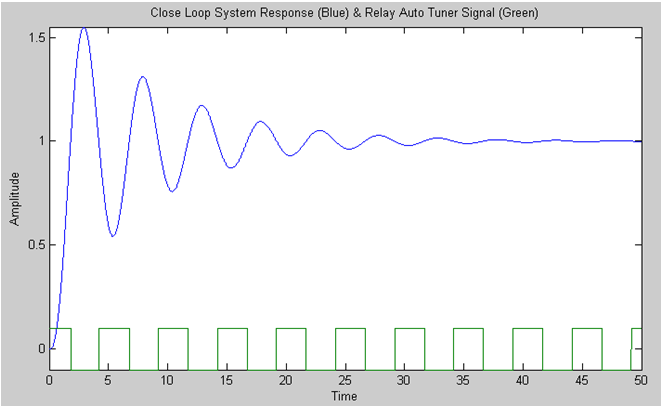Figure 6: Close loop system response when PI- controller is in action.

PID Controller:
The gain, integral time, and derivative time are 4.776, 1.865, and 0.466 respectively. In figure (7), the response curve obtained by using the gain, integral time and derivative time values.Figure 7: Close loop system response when PID- controller is in action.

By comparing the above responses due to the implementation of P, PI and PID controllers, the PID gives the finest result having the highest gain value of 4.776 among the three controllers. And, comparing figures 5 and 6, although only P controller shows better response than the PI which has a gain value of 3.98, but the DC gain is not 1.

2. When the plant transfer function is

For a=2:
The numerator and denominator of the transfer function are  and [1 4 5 2] respectively. The amplitude of the relay auto-tuner pulse signal h is 0.1 for which the system becomes unstable. The ultimate gain Kcu according to equation (1) is 17.45. (A=0.0073, Pu=2.87). Figure 8 shows the signal generated by the relay auto-tuner (green) and response from the plant (blue) which shows sustained oscillations.

Figure 8: Plot showing the close loop system dynamic response due to the relay input pulse.

P Controller:
The gain value is 8.73.Figure 9: Close loop system response when P- controller is in action.

PI Controller:
The gain is 7.853 and integral time is 2.392.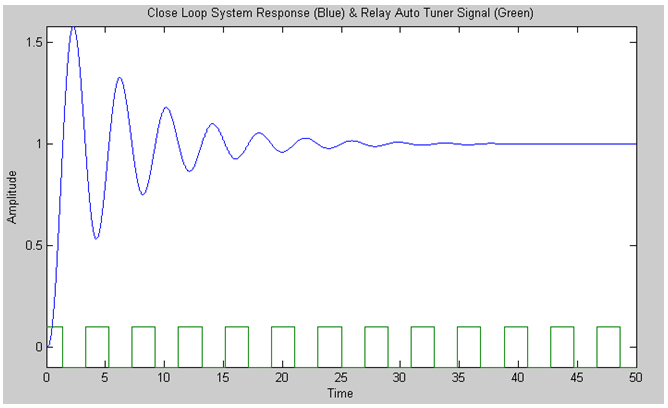Figure 10: Close loop system response when PI- controller is in action.

PID Controller:
The gain, integral time, and derivative time are 10.47, 1.435, and 0.359 respectively.Figure 11: Close loop system response when PID- controller is in action.

From figures 9, 10 and 11, using PID controller shows best performance among the three controllers. Although the overshoot in using PID is little higher than the P controller, nevertheless PID shows faster dynamic plant response than P controller. And, it is again noticeable that PID controller gain value 10.47 which is the highest among the others. PI controller which has the lowest gain 2.392 shows poor performance.

For a=10:
The numerator and denominator of the transfer function are  and [1 12 21 10] respectively. The amplitude of the relay auto-tuner pulse signal h is 0.1 for which the system becomes unstable. The ultimate gain Kcu according to equation (1) is 227.48. (A=0.00056, Pu=1.44). Figure 12 highlights the sustained oscillation of the plant due to the implementation of the relay auto-tuner.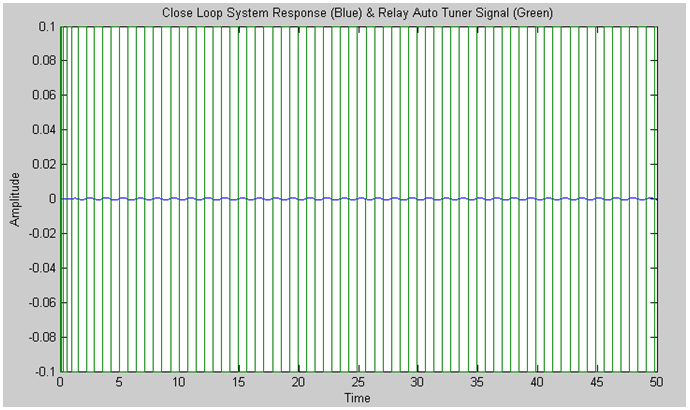Figure 12: Plot showing the close loop system dynamic response due to relay input.

P Controller:
The gain value is 113.74.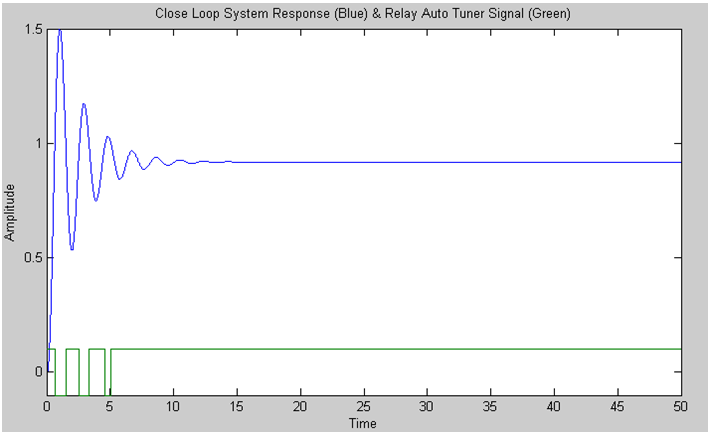Figure 13: Close loop system response when P- controller is in action.

PI Controller:
The gain is 102.366 and integral time is 1.2.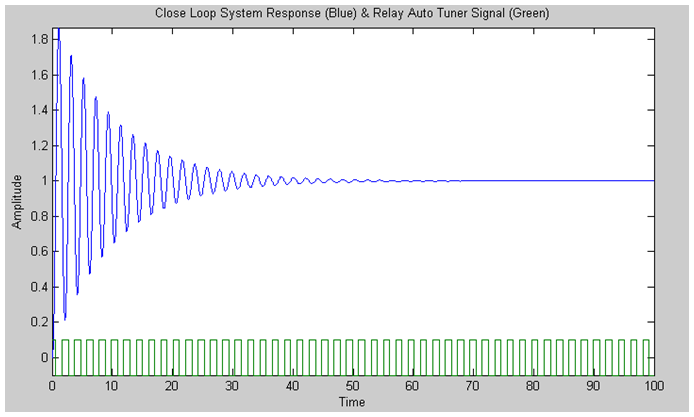Figure 14: Close loop system response when PI- controller is in action.

PID Controller:
The gain, integral time, and derivative time are 136.488, 0.72, and 0.18 respectively.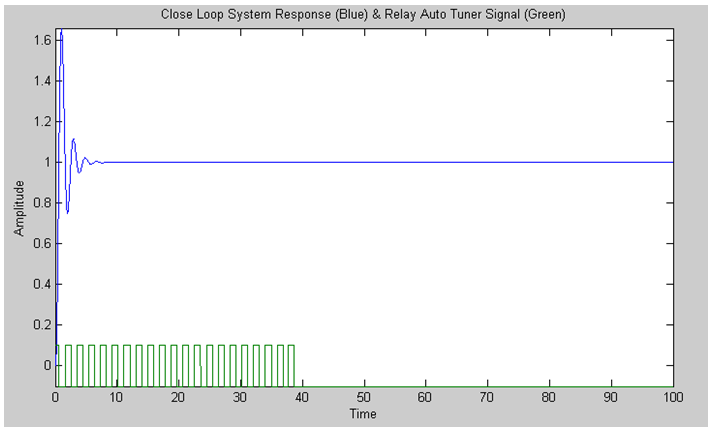Figure 15: Close loop system response when PID- controller is in action.

Analyzing above figures 13, 14 and 15, it is seen again the plant response obtained by the implementation of PID controller shows promising result which has the highest gain value 136.488. In case of the PI controller, the system is unstable during first 30 seconds (approximately) and then it gets settle down. The gain value of the PI is 102.366 which is the lowest among the three controllers.

For a=50:
The numerator and denominator of the transfer function are  and [1 52 101 50] respectively. The amplitude of the relay auto-tuner pulse signal h is 500 for which the system becomes unstable. The ultimate gain Kcu according to equation (1) is 5095.54. (A=0.000025, Pu=0.69). In this case, increasing the value of ‘a’ to 50 also increases the amplitude of the relay auto-tuner to 500 for which the system shows sustained oscillations. Following plot 16 shows the marginal stability of the system.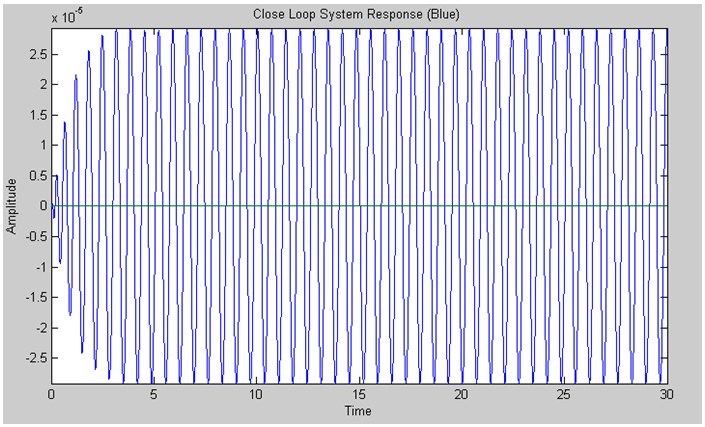Figure 16: Plot showing the close loop system dynamic response due to relay input.

P Controller:
The gain value is 2547.77.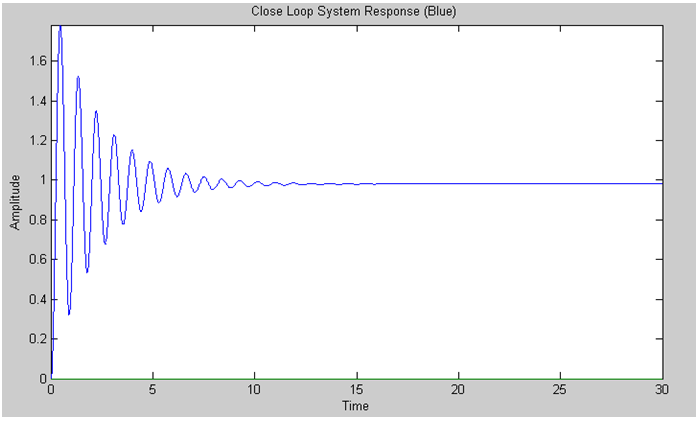Figure 17: Close loop system response when P- controller is in action.

PI Controller:
The gain is 2292.99 and integral time is 0.575.Figure 18: Close loop system response when PI- controller is in action.Figure 19: Close loop system response when integral time is set 2 seconds for the PI- controller.

PID Controller:
The gain, integral time, and derivative time are 3057.32, 0.345, and 0.086 respectively.Figure 20: Close loop system response when PID- controller is in action.

From the above figures 17, 18, 19 and 20, increasing the value of ‘a’ also increases the gain of each controllers. And, finally it is understood vividly that the response of the plant due to the implementation of PID controller is much better than the other two controllers. The application of PI controller in this case makes the system totally unstable (figure 18). To see further to get the system stability, integral time was increased to 2 seconds (figure 19) and after around 15 seconds the system settles down to magnitude 1.

3. When the plant transfer function isFigure 21: SIMULINK model for the system when a time delay block is added before the plant.

When τ is 0.5

The numerator and denominator of the transfer function are  and [1 1] respectively. The amplitude of the relay auto-tuner pulse signal h is 0.1 for which the system becomes unstable. The ultimate gain Kcu according to equation (1) is 3.27. (A=0.039, Pu=1.67). Here, a time delay is added before the plant which obviously makes the system dynamics little more complex than previous cases. Figure 21 shows the plant response with transport delay when relay auto-tuner is applied.Figure 21: Plot showing the close loop system sustained oscillation due to relay input.

P Controller:
The gain value is 1.63.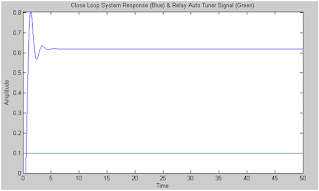Figure 22: Close loop system response when P- controller is in action.

PI Controller:
The gain is 1.47 and integral time is 1.39.Figure 23: Close loop system response when PI- controller is in action.

PID Controller:
The gain, integral time, and derivative time are 1.962, 0.835, and 0.209 respectively.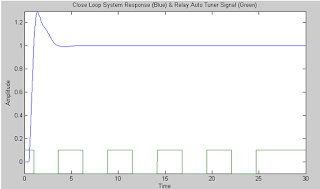Figure 24: Close loop system response when PID- controller is in action.

By examining figures 22, 23 and 24, implementing PI controller gives better performance than P controller in this case. But the gain value is less than gain of P. The DC gain of the plant is low in case of using P controller with respect to unit step input. The response of the plant is best while using PID controller which gets the highest gain value among the other controllers.

When τ is 1.0
The numerator and denominator of the transfer function are  and [1 1] respectively. The amplitude of the relay auto-tuner pulse signal h is 0.1 for which the system becomes unstable. The ultimate gain Kcu according to equation (1) is 2.022. (A=0.063, Pu=3). The sustained oscillation of the plant due to the relay auto-tuner application is shown by following figure 25.Figure 25: Plot showing the close loop system sustained oscillation due to relay input.

P Controller:
The gain value is 1.011.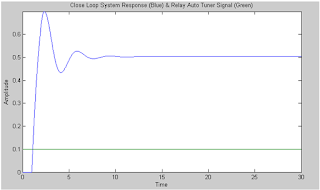Figure 26: Close loop system response when P- controller is in action.

PI Controller:
The gain is 0.9099 and integral time is 2.5.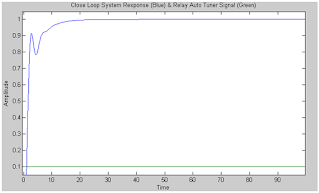Figure 27: Close loop system response when PI- controller is in action.

PID Controller:
The gain, integral time, and derivative time are 1.2132, 1.5, and 0.375 respectively.Figure 28: Close loop system response when PID- controller is in action.

Again by examining figures 26, 27 and 28, implementing PI controller gives better performance than P controller. However, the gain value is less than gain of P. The DC gain of the plant is low in case of using P controller with respect to unit step input which is around 0.5. The response of the plant is best while using PID controller which gets the highest gain value among the other controllers. It is also worth mentioning that increasing the time delay correspondingly decreases the gain of the controllers.

When τ is 3.0
The numerator and denominator of the transfer function are  and [1 1] respectively. The amplitude of the relay auto-tuner pulse signal h is 0.1 for which the system becomes unstable. The ultimate gain Kcu according to equation (1) is 1.36. (A=0.094, Pu=7.28). Figure 29 shows the plant response to the relay auto-tuner which behaves as marginally stable.Figure 29: Plot showing the close loop system sustained oscillation due to relay input.

P Controller:
The gain value is 0.678.Figure 30: Close loop system response when P- controller is in action.

PI Controller:
The gain is 0.612 and integral time is 6.067.Figure 31: Close loop system response when PI- controller is in action.

PID Controller:
The gain, integral time, and derivative time are 0.816, 3.64, and 0.91 respectively.Figure 32: Close loop system response when PID- controller is in action.

Finally by observing figures 30, 31 and 32, implementing PI controller gives better performance than P controller although the gain value is less than gain of P. The DC gain of the plant is low in case of using P controller with respect to unit step input which is around 0.4. The response of the plant is best while using PID controller which gets the highest gain value among the other controllers. Again, it is worth noticeable that increasing the time delay correspondingly decreases the gain of the controllers and further degrades the overall system response to the unit step input.

Part 02 (Implementation of the Relay Auto-tuner on Physical Plant)

Implementation of the Relay Auto-tuner on Water Tank System
Simulations have shown some beautiful aspects of implementing relay auto-tuner for tuning PID controller to obtain desired system performance. In this section, a relay auto-tuner is implemented to find the sustained oscillations of the physical water tank system. In EEEL-155 lab, a SIMULINK model is there for water tank level control. For this experiment, this model has been modified to accommodate the relay controller which is shown in figure 33. For the three configurations of the PID controller: P, PI and PID, experiments are carried out to understand and verify the Ziegler-Nichols’s PID tuning method.

The amplitude of the relay auto-tuner pulse signal h is 10 for which the water tank system becomes marginally stable. The ultimate gain Kcu according to equation (1) is 25.52. (A=0.499, Pu=2.43)

Following figure 34 highlights the close loop system response and the relay auto-tuner response from which the ultimate gain is determined. A manual switch is implemented to switch from relay auto-tuner mode to linear control mode (figure 33).Figure 33: SIMULINK model to study the effect of relay auto-tuner on physical water tank experimental test rig.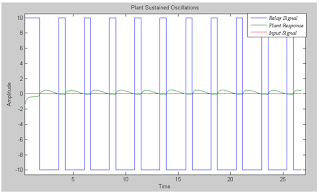Figure 34: Plot showing the close loop system dynamic response due to the relay input pulse.

P Controller:
The gain value is 12.76.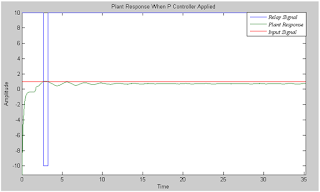Figure 35: Water tank close loop system response when P controller is implemented.

PI Controller:
The gain is 11.48 and integral time is 2.03.Figure 36: Water tank close loop system response when PI controller is implemented.

PID Controller:
The gain, integral time, and derivative time are 15.31, 1.22, and 0.3 respectively.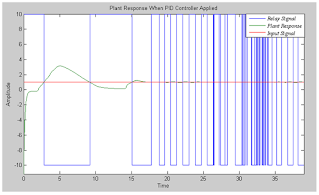Figure 37: Water tank close loop system response when PID controller is implemented.

By observing the figures 35, 36 and 37, it can be stated that the tuning method by Ziegler-Nichols works well not only in simulations but also in experiment in a qualitative sense. Using PI and PID controllers with the water tank system achieve DC gain 1 after some oscillations whereas only P controller fails to achieve the gain 1. Nonetheless, implementing P controller decreases overshoots which are seen in case of PI and PID controllers. At the end of this report, it is worth to be noted that the results obtained in the experiment could be much better by further tuning the PID controller parameters as in this case the parameters are taken only based on the Ziegler-Nichols empirical formulae which are sometimes inefficient for non-linear systems.

References

 Kutsuhiko Ogata, Modern Control Engineering, fifth edition, Pearson Education, Inc., publishing as Prentice Hall, 2010.

 Antonio Flores T., Relay Feedback Auto Tuning of PID Controllers, October 4, 2007.

#Blog #Blogger #Relay #AutoTuning #Matlab #Simulink #PIDcontrol #ZieglerNichols

1.what is the Pu ?

1.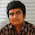Pu refers to the oscillation period of the close loop system

2.Could you demonstrate how to set up the relay block to produce pulse like above?

3.Can you explain how to set up the relay block please?

1.You can find the relay block from the Simulink library. Based on your model, you need to adjust the parameters in the relay block.

2.Do you have any guide on how to adjust the relay?

3.I meant how do you know the turn on point and turn off point?

4.Hi, thanks for your question. You need to test with different parameters for the relay. For example, for this specific article, relay is used to tune the PID controller. So, it depends on the application or your designed system. I am not certain if there is any general guide on turning on/off the relay.

4.Hi, how did you tune your PID parameters, if you used the code can you please share it?